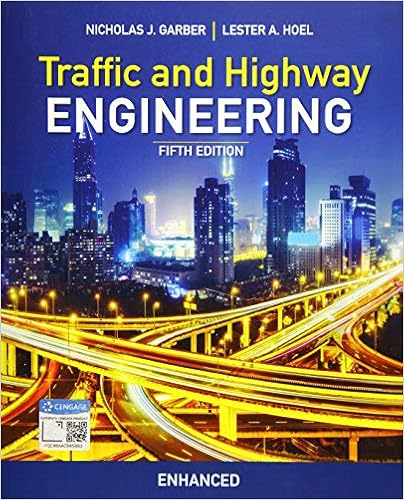# caclculus unit 7.docx - George Case MCV4UB 17124053...

• Homework Help
• 13
• 100% (8) 8 out of 8 people found this document helpful

This preview shows page 1 - 5 out of 13 pages.

##### We have textbook solutions for you!
The document you are viewing contains questions related to this textbook.The document you are viewing contains questions related to this textbook.
Chapter 7 / Exercise 7-2
Traffic and Highway Engineering, Enhanced Edition
Garber/HoelExpert Verified
George Case MCV4UB 17124053 Assessment of Learning Unit 7 Lesson 20 Task 1 1. Determine the angle between each of the following pairs of vectors a. u = ( 2, 4 ) v =( 1, 3 ) °
##### We have textbook solutions for you!
The document you are viewing contains questions related to this textbook.The document you are viewing contains questions related to this textbook.
Chapter 7 / Exercise 7-2
Traffic and Highway Engineering, Enhanced Edition
Garber/HoelExpert Verified
George Case MCV4UB 17124053 5 ¿ 2 4 ¿ 2 + ¿ 1 ¿ 2 + ¿ ¿ | p | = ¿ | p | = 1 + 16 + 25 | p | = 42 3 ¿ ¿ 1 ¿ 2 + ¿ 3 ¿ 2 + ¿ ¿ | q | = ¿ | q | = 9 + 1 + 9 | q | = 19 cosθ = 8 ( 42 )( 19 ) cosθ = 8 798 cosθ = 0.2832 θ = cos 1 0.2832 θ = 73.55 ° Therefore the angle between p qis 73.55 ° 2. Find the slope of the vector that is perpendicular to the scalar equation
3. Write an alternate vector equation for the following line. Change both the point and the direction vector: w = ( 4, 1,3 ) + t (− 2,1,7 Sub real number for t. In this case sub t for 4 )
George Case MCV4UB 17124053 ( x, y ,z ) = ( 4, 1,3 ) + 4 (− 2,1,7 ) ( x, y ,z ) = ( 4, 1,3 ) +(− 8,4,28 ) ( x, y ,z ) =( 4 8, 1 + 4,3 + 28 ) ( x, y ,z ) =(− 4,3,31 ) Determine another direction vector using scalar multiple of t (− 2,1,7 )
4. Determine whether the angle between each of the following pairs of vectors is acute, obtuse or neither a. a = ( 10, 4,1 ) b =( 3, 2,2 )
5. Given the vector equation of a line in 2-space ( x, y ) = ( 3,2 ) + t ( 2,4 ) write a scalar equation for the line The equation gives a point on the line (3,2) 0
6. Write a vector equation for the line that passes through the point
George Case MCV4UB 17124053 x z valuesmust equal 0 y cantake any value ( x, y ,z ) = ( 1,0,3 ) + t ( 0,4,0 ) This is a vector equation passing through the given point and parallel to the y axis.
•••